Courses

# Test: Electronic Devices - 4

## 10 Questions MCQ Test RRB JE for Electronics & Communication Engineering | Test: Electronic Devices - 4

Description
This mock test of Test: Electronic Devices - 4 for Electronics and Communication Engineering (ECE) helps you for every Electronics and Communication Engineering (ECE) entrance exam. This contains 10 Multiple Choice Questions for Electronics and Communication Engineering (ECE) Test: Electronic Devices - 4 (mcq) to study with solutions a complete question bank. The solved questions answers in this Test: Electronic Devices - 4 quiz give you a good mix of easy questions and tough questions. Electronics and Communication Engineering (ECE) students definitely take this Test: Electronic Devices - 4 exercise for a better result in the exam. You can find other Test: Electronic Devices - 4 extra questions, long questions & short questions for Electronics and Communication Engineering (ECE) on EduRev as well by searching above.
QUESTION: 1

### For a single stage BJT common base amplifier.

Solution: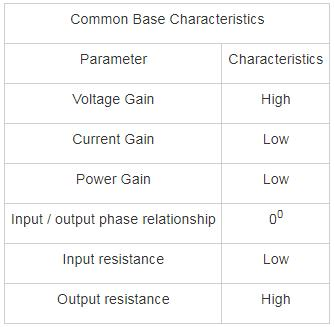∴ Voltage gain can be greater than unity but current gain is always less than unity.

*Answer can only contain numeric values
QUESTION: 2

### A bipolar transistor has an emitter current of 1 mA. The emitter injection efficiency is 0.99, base transit factor is 0.995 and depletion region recombination factor of 0.998. The base current flowing through the transistor is _____μA.

Solution:

The common emitter current gain is given by:

α = γ ∗ β ∗ δ

= 0.99 × 0.995 × 0.998 = 0.983

Collector current

Ic = αIE = 0.983 mA

Base current

IB = IE – IC

= 17 μA

*Answer can only contain numeric values
QUESTION: 3

### In a Bi-polar junction transistor the base width is 0.54 μm and base diffusion constant is DB = 25 cm2/sec. The Base transit time time is ________ × 10-10 sec

Solution:

The Base transit time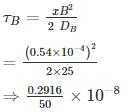⇒ 0.5832 × 10-10

*Answer can only contain numeric values
QUESTION: 4

Find the value of bias resistor (in kΩ) if quiescent collector current and voltage value are 4.6 mA and 2.2 V. The transistor has DC gain 110, VBE = 0.7 V and VCC = 4.5 V.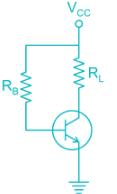Solution:

Applying KVL in collector to emitter loop

VCC = RLIC + VCE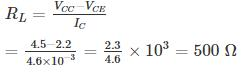Now applying KVL in collector to base loop

IBRB + VBE = VCC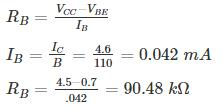QUESTION: 5

Consider two pnp bipolar junction transistors. For the first transistor when emitter to collector voltage is 5 V, VEB is 0.85 V and emitter current is 10 A. (The β for this transistor is 15). Second BJT conducts with a collector current of 1 mA and VEB = 0.70. The ratio of emitter-base junction area of the first transistor to the second transistor is ______.

(Assume KT = 26 mV)

Solution:

Given: VEC = 5V-, this means pnp transistor is operating in the active node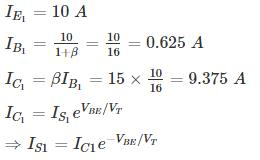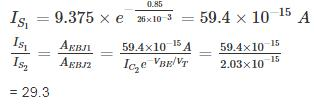*Answer can only contain numeric values
QUESTION: 6

The leakage current of a transistor with usual notation are ICEO = 410 μA, ICBO = 5 μA, and IB = 30μA. Calculate the IC ________mA

Solution:

ICEO = 410 μA, ICBO = 5 μA, IB = 30 μA

IC­ = βIB + (1 + β) ICBO

ICEO = (1 + β) ICBO

410 = (1 + β)5

β = 81

IC = 81 × 30 + 82 × 5

IC = 2.84 mA

QUESTION: 7

For what value of current gain β , the given transistor will be in saturation

(Assume Vin = 5V, VBE(SAT) = 0.8 V , VCE(SAT) = 0.2V)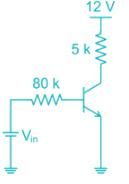Solution:

Apply kVL in base emitter loop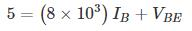at saturation VBE(sat) = 0.8

substituting the value of VBE

IB = 0.0525 mA

Apply Kvl , Form the collector to emitter

5 × 103 × I+ VCE = 12 V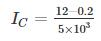= 2.36 mA

At the edge of saturation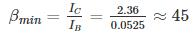Hence for β value greater than 45 the transistor will be in saturation

*Answer can only contain numeric values
QUESTION: 8

An npn bipolar transistor having uniform doping of NE = 1018 cm-3 NB = 1016 cm-3 and NC = 6 × 1015 cm-3 is operating in the inverse-active mode with VBE = -2V and VBC = 0.6 V. The geometry of transistor is shown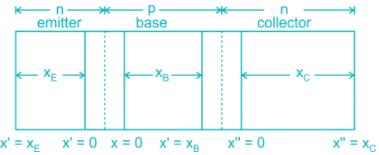The minority carrier concentration at x = xB is _____ × 1014 cm-3

(Assume n = 1.5 × 1010/cm3, Vt ­= 25 mV)

Solution: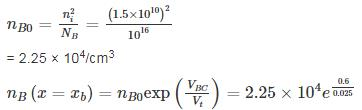= 5.96 × 1014 cm-3

QUESTION: 9

The common emitter forward current gain of the transistor shown is β = 100
The transistor is operating inSolution: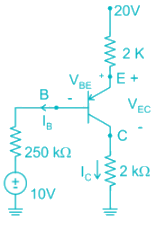Assume Transistor in Active region

20 – 2 (IB + IC) – 0.7 – 250 IB – 10 = 0

20 – 2 × 101 IB – 0.7 – 250 IB – 10 = 0

10 – 452 IB – 0.7 = 0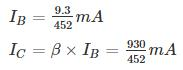20 – 2 (IB + IC) - VEC – 2IC = 0

20 – 202 IB - VEC = 0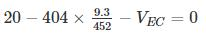VEC = 11.68 V

VCB = VCE + VEB = -VEC + VEB = - 11.68 + .7

VCB = -10.98

∴ Collector base junction is Reverse Biased

∴ Transistor is operating in forward active region

Second Method

Assume transistor in saturation region

IB min ≤ IB

i) VEB (sat) = 0.8 V

ii) VEC (sat) = 0.2 V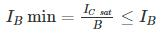IC ≠ βIB ----- Because it is valid only active region

20 – 2 (IB + IC) – 0.8 - 250 IB – 10 = 0

20 – 2 IB – 2IC – 0.8 – 250 IB – 10 = 0

10 – 252 IB – 2IC – 0.8 = 0

252 IB + 2I= 9.2      -------- (1)

20 – 2 (IB + IC) – VEC(sat) – 2IC = 0

20 – 2IB – 2IC – 0.2 – 2IC = 19.8      -------- (2)

By solving equation (1) and (2)

IB = 0.00278 mA

IC = 4.95 mA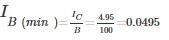∴ IB(min) > IB

∴ Transistor is operating in forward active region

QUESTION: 10

In a silicon PNP transistor the mobility of charge carries is μ4 = 1300 cm2/V-s and μp = 450 cm2/V-s and carrier life time τp = 0.10 μs. The most appropriate base width for effective transistor function is (Take T = 300° k)

Solution:

Given μn = 1300 cm2/V-s and μp = 450 cm2/V-s

Dp = 450 × 0.0259

= 11.655 cm2/s

Base diffusion length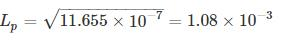= 1.08 mm

For injected holes from emitter to reach the collector they should not be recombined in base. Hence the base width should be very less compared to base diffusion length.

W ≪ LP

In all options the base width is significant compared to base diffusion length.